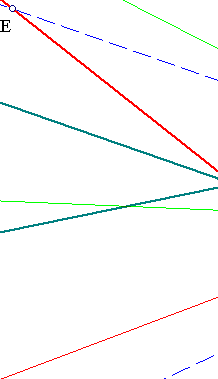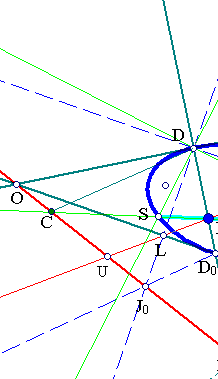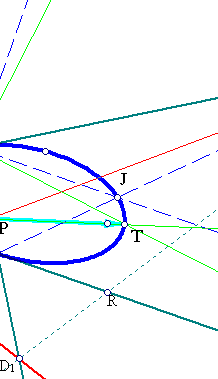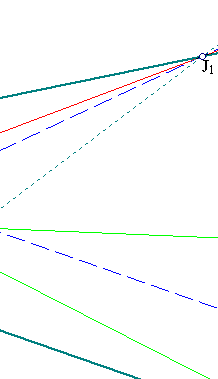##Fregier involution by orthogonals from a conic-point

Let D be a point on a conic and consider the bundle of all lines passing through D. Consider the map of the conic to itself, which to each point S, corresponds the point T = F(S), such that line [DS] is orthogonal to the line [DT]. F is an involutive homography and defines a point P (Fregier point of the involution) such that all lines [ST] pass through P.The proof follows from the general properties of involutions (see InvolutiveHomography.html ). It suffices to show that F, defined by the previous recipe is an homography. But this is seen at once by taking a good parametrization through a line parallel to the tangent at D. Setting the origin of the line-coordinates to the intersection point of this line with the normal at D, the relation between the coordinates of S and T becomes x*x'=k, k being a constant. Thus the relation is indeed involutive and the property follows from the characteristic property of such maps, to have the lines through {X,F(X)} passing through a fixed point.
The following arguments identify this involution with one which constructs explicitly P and its polar (line e=OD1)):
 Consider first the tangent line and the normal of the conic at D. Let O be the intersection-point of the tangents at D and D0, which is the intersection point of the normal at D with the conic, other than D.
 From D draw a parallel to the tangent [OD0], cutting the conic at point J. Let J1, J0 be respectivelly the intersection points of line JD0 with DO and the orthogonal to DJ at D respectively. Let D1 be the intersection of DD0 and OJ0.
 Let L be the intersection point of lines [OD0] and [DJ0]. Line [LJ1] intersects lines [DD1], [OD1] respectively at points P, U, which are harmonic conjugate to L, J1 (quadilateral ODD0J0).
 Points P, D1 are also harmonic conjugate w.r. to D, D0 (same quadrilateral). Thus O and D1 being on the polar of P implies that later coincides with line [OD1].
 It follows that for every point C on line OD1, line [CP] intersects the conic at points S, T and the correspondance T = F(S) defines an involution of the conic, with corresponding Fregier point P.
 From its definition D0 = F(D) and the involution introduced in the corresponding line-bundle at D interchanges two orthogonal lines: [DD0] and [DJ1]. Hence all the lines of the line bundle are interchanged, by the involution, in pairs of lines at right angles ( exercise: Pedoe, a course of Geometry, exercise 80.1, p. 355 ).
 To solve this exercise parametrize the bundle through a line orthogonal to the bisector of the two lines at right angle, with origin at the intersection point with the bisector. In this system the parameters of the lines in involution satisfy an equation of the form Axx'+B(x+x')+C=0. For the orthogonal lines we have x'=-x=r (constant) hence C-r2A=0 and the equation becomes A(xx'+r2)+B(x+x')=0. This is only possible if B=0 and xx'=-r2, which proves the exercise.
See FregierPolar.html for another easy way to construct point P and its corresponding polar line.